## 6) Bonds

Bonds of 5: Building a Wall

### Mathematics

Develop the concept of:

• The whole of 5 being equal to two parts joined together.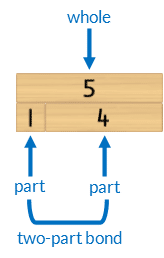The two parts bond (join) together to become equal to the length of the whole.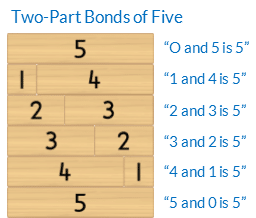• The Commutative Property of Addition: swapping the order of the parts does not alter the size of the whole. For example, changing the order of the parts of 3 and 2  to 2 and 3 does not alter the size of the whole.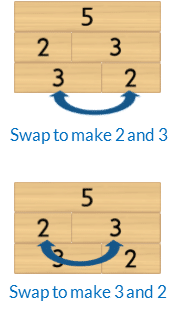• Mathematics as the science of pattern.

### Language

• “(Part) and (part) is (whole)”. eg “4 and 1 is 5”
• addition as “and” when joining parts
• equals as “is”, “is equal to”
• bond
• too long, too big, too short, too small
• commutative property
• row (horizontal)

### Core Activity Support MaterialsClick to open in a new tab.## Differentiation

### A little easier

##### Scaffold finding the Bonds of 5 in counting order
• Place the 5 block horizontally in front of the student.
• Then place the 1 block below the 5. Ask the student, “Which number joins with 1 to make it the same length as 5?” Model saying the bond, whilst touching the related blocks, “Yes, 1 and 4 is 5”.
• Repeat this process with each block from 2 to 5 until the whole wall is formed.
##### Scaffold discussing the commutative property of addition
• Focus on one two-part bond at a time. Use the 5 block as the whole.
• Begin with the bond of 1 an 4. Place both rows of related two-part bonds beneath the whole. Then rearrange the parts within each row to make their order the same.• Repeat for the bond of 2 and 3.### A little harder

##### Develop fluency recalling two-part bonds of five
• The student builds a wall of five that is not in consecutive order.
• One block from each row is removed whilst the student closes their eyes.
• The student identifies the missing block in each row.##### Three-part bonds of five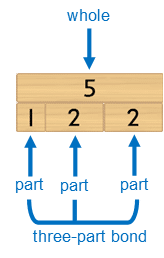• Build each row with three blocks.
• Identify which two-part bonds are similar to related three-part bonds. For example, 2 and 3 can be partitioned to become 1+1+3.
• Rearrange the three-part bonds to reinforce the commutative property. For example 1+1+3 is equal to 1+3+1 and 3+1+1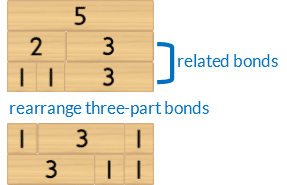### Progression

Develop fluency building two-part bonds of five with the next activity. Go to

##### Activity 7

Bonds of 5: Fluency, Filling A Wall